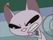cancel
Showing results for
Did you mean:Frequent Visitor

## Replicating excel formula | precedent referencing

Hello community,

Need help in replicating the following formula in DAX or in power query -

In Excel we can easily use precedent referencing but in dax or power query I haven't found anything, i need ut for below use, in first screenshot it's the evaluated values, and in second, formulas behind those, i just need a way i can do the same in power bi at the end,

note that first values are manually entered '0'copy paste the values from below to test out

 Total engine hours Pulse 0 0 0.8 0.8 1.65 1.65 0.3 1.65 0.3 1.65 6.1 7.45 1.15 7.45
1 ACCEPTED SOLUTIONMemorable Member
``````let
Source = your_source_table,
data = List.Buffer(Source[Total engine hours]),
max_count = List.Count(data),
pulse =
List.Generate(
() => [i = 0, p = 0],
(x) => x[i] < max_count,
(x) => [i = x[i] + 1, p = if (data{i} >= data{x[i]}) then x[p] + data{i} - data{x[i]} else x[p]],
(x) => x[p]
),
out = Table.FromColumns(Table.ToColumns(Source) & {pulse}, Table.ColumnNames(Source) & {"Pulse"})
in
out``````
6 REPLIES 6Memorable Member
``````let
Source = Excel.CurrentWorkbook(){[Name="Table1"]}[Content],
data = List.Buffer(Source[Total engine hours]),
max_count = List.Count(data),
pulse =
List.Generate(
() => [i = 0, p = 0],
(x) => x[i] < max_count,
(x) => [i = x[i] + 1, p = if (data{i} >= data{x[i]}) then x[p] + data{i} - data{x[i]} else x[p]],
(x) => x[p]
),
out = Table.FromColumns({data, pulse}, {"Total engine hours", "Pulse"})
in
out``````Frequent Visitor

@AlienSx, this sort of solves my issue, but my actual data comes from sql database which has this 'Total engine hours' with multiple other columns, which of the bits i would need to edit then?Memorable Member
``````let
Source = your_source_table,
data = List.Buffer(Source[Total engine hours]),
max_count = List.Count(data),
pulse =
List.Generate(
() => [i = 0, p = 0],
(x) => x[i] < max_count,
(x) => [i = x[i] + 1, p = if (data{i} >= data{x[i]}) then x[p] + data{i} - data{x[i]} else x[p]],
(x) => x[p]
),
out = Table.FromColumns(Table.ToColumns(Source) & {pulse}, Table.ColumnNames(Source) & {"Pulse"})
in
out``````Frequent Visitor

I believe this will definitely help, thank you so much!

Do you know if there's a way we can achieve the same through DAX?Memorable Member

DAX - most likely yes but I am not a DAX guy (yet) 😎Frequent Visitor

Pretty sure you will reach there very soon, really appreciate your help!